# Distances

There are a number of distance-related computations, measures, and indices that can prove useful in your game programming.

#### Distance Between Two Points

The easiest way to compute the distance between two points is to use the Euclidean distance metric. Given P and Q in a 3D world, the distance is computed by: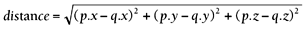Computing square roots can be costly. Moreover, in some cases (such as spatial sorting by distance), you will not need the distance itself, but just a way to measure objects according to their distance. In this case, you can override the square root and use the expression:

` distance_squared = (p.x q.x)2+(p.y q.y)2+(p.z q.z)2 `

A third method is to use Manhattan distance metrics, which are defined as:

` distance_manhattan = abs(p.x q.x)+abs(p.y-q.y)+abs(p.z-q.z) `

Manhattan distances are the 0-level Euclidean norm, defined by the general expression: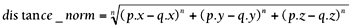Manhattan distances are used as very fast pseudodistances, especially to cull away distant objects. It is a very cheap test that can help us discard irrelevant primitives (both in terms of logic and presentation).

#### Distance Between Two Lines

Given two lines R and S in (origin, direction vector) form, we can compute the minimum distance between them easily. The lines have the following expression:

` R: `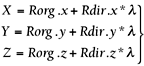` S: `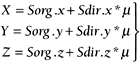These are the parametric equations of the lines, and they are very easy to construct from game data structures. The distance between them is computed with the following: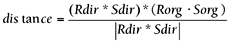This formula can be somehow sped up. For crossing lines, the numerator will always be zero, and thus we can save the division and modulo operations in the denominator.

#### Distance from a Point to a Line

The distance from a point P to a line in parametric form (defined by Org and Dir) can easily be computed with the following: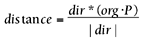By storing a normalized direction vector, we can save the divide, thus speeding up the computation.

#### Distance from a Point to a Plane

Distance from a point P to a plane in 3D space can be computed easily using the normal distance to origin plane representation. A plane can be represented as:

` N.x*X+N.y*Y+N.z*Z+D=0 `

where N is the normal to the plane and D is the minimum distance from the plane to the origin. Assuming this representation, the distance is computed as follows: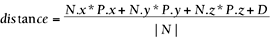As usual, keeping the plane's normal as a unit vector speeds the formula, so we can save the modulo and division in the denominator.

#### Distance Between Two Planes

Finding the distance between two planes is pretty straightforward:

1. Test if the two normal vectors are the same; if they are, the planes are parallel. If they are not, distance is 0 (they intersect).

2. Select a point in one of the planes.

3. Perform point-plane distance as shown earlier using that point and the opposite plane.Core Techniques and Algorithms in Game Programming2003
ISBN: N/A
EAN: N/A
Year: 2004
Pages: 261